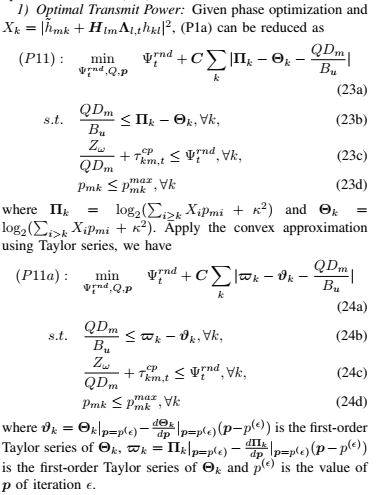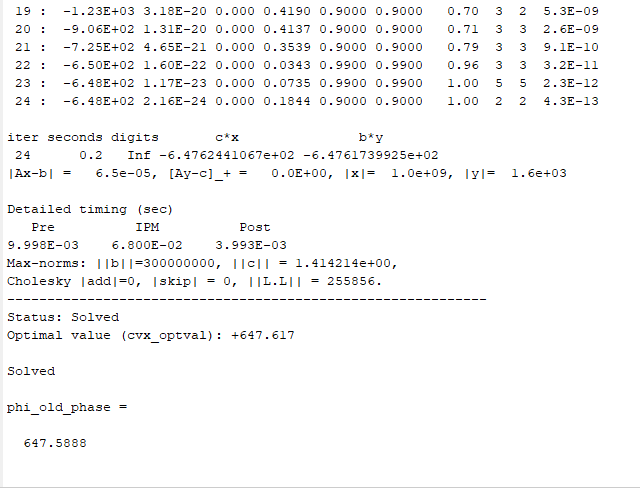# Which solver should I choose

Hi everyone,
I have problem like thisI tried to use different solvers to check. And even each solver (sedumi and ecos) has the output of solved but the result is different. And more thing is that for ecos, the result is not correct for constraint. So I want to know how to choose the solver to solve problem. This is my cvx code where the data input is the same and variable are \phi and Q
Lambda=diag(s_n);
X1=(h_mi’+(H_lmLambda)(h_kl’));%1xK
X=((abs(X1).^2))’ ;
no_iteration_power=50;
gap_p=0.0001;

for lp=1:no_iteration_power
cvx_begin  quiet
cvx_precision high
cvx_solver sedumi;
variables phi Q
variables p_mi(K,1)
expression Y(K,1)
for k=1:1:K
Zkn=X(k:K,:).'*p_mi_n(k:K,1);%%%k:K
Zk1n=Zkn-X(k,:)*p_mi_n(k,1);%%%k+1:K
Y(k,:)=-log(Zk1n+kappa)-(X(k+1:K,:).'*(p_mi(k+1:K,:)-p_mi_n(k+1:K,:)))/(Zk1n+kappa)...
+log(Zkn+kappa)+(X(k:K,:).'*(p_mi(k:K,:)-p_mi_n(k:K,:)))/(Zkn+kappa);
end

minimize(phi+C*sum(abs(-Y+log(2)*(Q*D1/B_u))))
subject to
log(2)*(Q*D1/B_u) <=Y;
D*inv_pos(D1*Q) +comp_latency <= phi;
p_mi<=p_mi_max;
p_mi>=p_mi_min;
0<=phi;
0<=Q;

cvx_end
disp(cvx_status)
if((sum(abs(Y-log(2)*(Q*D1/B_u))) <=gap_p)&&((phi-phi_old_p)<gap_p))
break;
else
p_mi_n=p_mi;
phi_old_p=phi;
end


end

  p_mi_n=p_mi;
phi_old_phase=phi
Q*D1


The result of phi is 647 in sedumi while the result is phi is 0.34 in ecos.

Try MOSEK. And also share the log outputs if you would like help regarding numerical issues (remove quiet)

thank you for your reply. This is the log output of ecos, sedumi and mosekI still question why with ecos, the ouput is solved eventhough the result violates the constraint (23c)/(24c). The comp_latency is [86.3066;39.9099;145.8018;43.1674;92.1499;36.9933];

Accorging to the Mosek (nrm) and Sedumi (|x|) log the solution has huge norm which may explain all kinds of numerical issues with the solvers. Ecos gives up. You could also try Mosek 9.2 instead of Mosek 8 you are using now. And rescale the problem so that the expected solution is not so big.

Thank you for your suggestion. After checking, the current mosek already is 9.2. ( I checked to uninstall). And there is one more thing I want to ask about norm as u said. Can you give me more detail for suggestion, such as how much for scale and how much for norm that we can accept. Thank you again

Your question is hard to answer. You should think about why norm is large and then change the model so it becomes smaller.

The large norm indicates you have an unstable model and it is unlikely you can trust it.

I can just add for completeness that the log output you posted is 100% from Mosek 8. Maybe your cvx is picking another Mosek from many installations than you think.

cvx_solver will show all the versions of Mosek available to CVX. You can specify the particular one you want.

If you are using CVX 2.1, it comes bundled with a Mosek 8.x. CVX 2.2 comes bundled with a Mosek 9.1. By default, CVX will choose the version of Mosek supplied with CVX even though you may have another version of Mosek installed elsewhere.

If you want your non-CVX-supplied version of Mosek to be the first on the list (and picked by default), you can rename or delete the mosek directory installed under the top level CVX directory, which will ensure that CVX doesn’t see it. Or if you always want to use Mosek as solver, just specify the version you want using cvx_solver, then cvx_save_prefs to save it across sessions.

Yes Thank you and sorry. I installed mosek 9.2 seperately with cvx in advance. Then mosek in cvx folder is 8.0 as you said. I misunderstood it.

Thank you for your reply. I am using cvx beta 3.x. Because Michal suggested me to use mosek 9.2, i already installed separately in matlab and it is said ok for matlab ( i tested by mosekdiag). However, I do not know how to use mosek 9.2 with cvx. Following your suggestion, I delete the mosek folder on the cvx folder and run cvx_setup again. Then cvx said mosek solver can not found. So to use mosek 9.2, is there only one way that i delete the current cvx and install cvx 2.2? Thank you

First of all, use CVX 2.2 instead of CVX 3.0beta, which is full of bugs.

if you installed Mosek 9.2 under MATLAB and mosekdiag says it is working, then when you install CVX, CVX should find it. Use cvx_solver to see the various versions of Mosek, then again to speoifty the specific version you want,

Thank you for your reply. I removed cvx3.0 beta and installed cvx 2.2 with mosek 9.1. Even I have mosek 9.2 under matlab, i can not see several version of mosek when i use cvx_solver. I donot know which I missed.
But the more important thing is that, I need to run my code in iteration way. The reason is that the original problem is non-convex, so I used Taylor approximation to approximate to convex problem ( problem p11a as my first post). As you know that, in the Taylor approximation, we need the value of variable in the previous iteration (p^{epsilon}) or the initial value of variable. That is why my code needs to run in the iteration way in the objective function is converges.

However, sometimes code works with many results in each iteration ( solved, unbounded…) then the final result is solved, that makes me do not know that the result is correct or not. Sometimes, it has the error :" Invalid constraint: {real affine} <= {complex affine}" for the log(2)(QD1/B_u) <=Y;. So could you please help me how to deal with these problem. What should I modify the code and/or any advise/suggestion for inital value that my code can run the iterative way and converges.

I hope you can understand my situation and help me. Thank you so much. I am new in cvx and also hope to learn from you.

My suggestion is to use a non-convex optimization solver, for instance under YALMIP. Whether that is acceptable to your advisor is between you.

Thank you so much. I will try to install Yalmip as your suggestion. But because we needs algorithm is time consuming effective, especially when the size of the variable becomes big ( i.e., the number of mobile users…)., I also want to learn about the convex optimization. So can you help me one more thing? is my above code is correct? why did i get the error " Invalid constraint: {real affine} <= {complex affine}" for the log(2)(Q*D1/B_u) <=Y? Thank you so much.

Apparently CVX thinks Y is complex. Is any of the input data to Y non-real?

Yes. It is complex because s_n is complex

inequality constraints, on the other hand, must be real.

It’s your model, not mine, so I’ll leave it to you how to fix it.

Ah. Yes. Thank you so much.